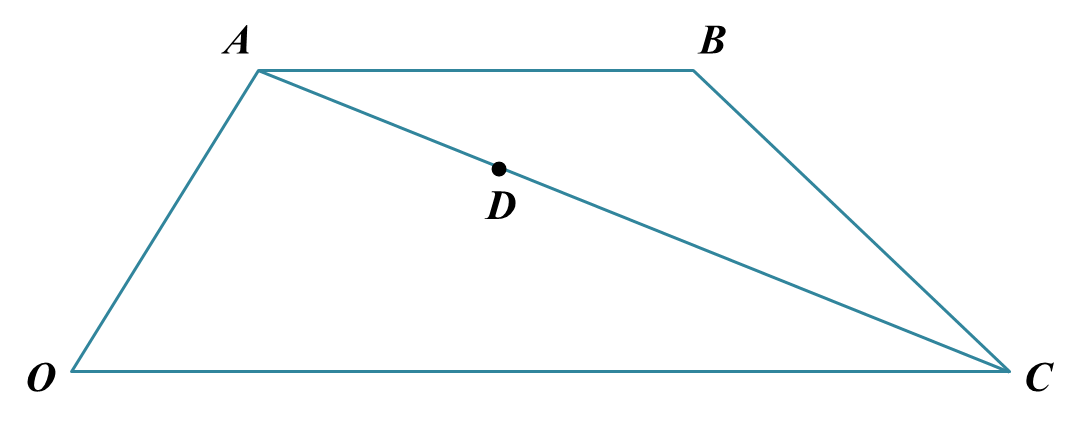# 4.7.3 Vectors, SPM Practice (Question 5 & 6)

Question 5:
Diagram below shows a triangle KLM.Solution:
(a)(i)

(a)(ii)

(b)

(c)

Question 6:
Diagram below shows a trapezium OABC and point D lies on AC.Solution
:

(a)(i)

(a)(ii)
$\begin{array}{l}\stackrel{\to }{OC}=2\stackrel{\to }{AB}\\ 18\underset{˜}{b}=2\left(\stackrel{\to }{AO}+\stackrel{\to }{OB}\right)\\ 18\underset{˜}{b}=2\left(-6\underset{˜}{a}+\stackrel{\to }{OB}\right)\\ 18\underset{˜}{b}=-12\underset{˜}{a}+2\stackrel{\to }{OB}\\ \stackrel{\to }{OB}=6\underset{˜}{a}+9\underset{˜}{b}\end{array}$

(b)